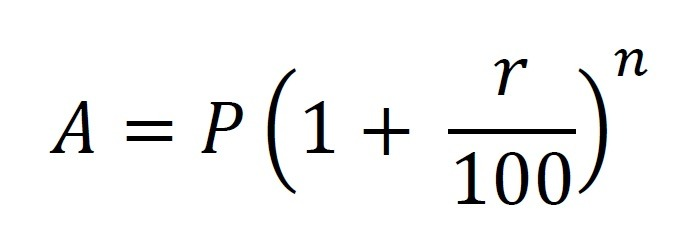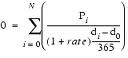Investor use variety of to maths tools to analysis the performance of their investment. We have taken some most useful ones and explain their purpose and how to use these calculator for finding your investment returns. The investment calculator allows you to determine the current & future value of your investment.

1.  Compound Interest

I want to take a loan of Rs. 200,000 to buy a used car. How much will the car cost me at annual interest rate of 9 %  for four (4) years.

The compound interest formula can be used here to calculate the final cost, which would include the loan amount and the interest paid.

The amount that is actually paid for Rs 2 lakh is Rs. 2,82,316. The total amount of interest charged for borrowing Rs. 2 lakh is 82316.

Formula : Future Value =  P(1 + R)^NP : Loan Amount    R : Rate of Interest   N: time in years

2.  Compound Annualized Growth Rate  – (CAGR)

I had invested Rs. 1 lakh in a mutual fund three year back at an NAV of 16. Now the NAV is Rs. 54. How should I calculate my returns on an annual basis?

Compound annualized growth rate (CAGR) will be used here to calculate the growth over a period of time. The gain of Rs. 38  (54-16) over 3 years on the initial NAV of Rs. 16 is simple return 238%  ((54-16)*100). However, it should not be construed as 48% average return over 3 years.

Formula :    CAGR = (((M/I)^(1/N))-1)*100CAGR  = (((54/16)^(1/3))-1)*100

CAGR  =  27.54%

M : Maturity Value   I : Initial Value  N : time in year

3.  Internal Rate of Return  – (IRR)

I paid Rs 18,572 every year on a money back insurance policy bought 20 year back. Every fifth year, I received Rs. 40,000 and Rs. 4.5 lakh on maturity. What was my rate of returns?

The internal rate of return (IRR) has to be calculated here.It is the interest rate accrued on an investment that has outflows and inflows at the same regular periods.

In the Excel page type Premium Rs. 18,572 as a negative figure -18572 (Outflow)

Maturity Amount & Money back type positive figure 40,000& 4,50,000  (Inflow)

Formula :    = IRR(E6:E26)

IRR :        5.28%

Also use for :  checking your Endowment policy.

4.   XIRR

I bought 500 shares on 1 Jan 2012 at Rs. 220, 100 share on 20 Mar 2012 at Rs. 185 and 50 share at Rs. 165 on 6 Jun 2012 , I sold all the 650 shares at Rs. 275 on 10 Mar 2013. What is the return on my investment ?

XIRR is used to determine the IRR when the outflows and inflows are at different periods. Calculation is similar  IRR’s. Only add Transaction date.

In an excel sheet type out data as shown here.

Outflow figures are negative (Purchase)  and inflow figures (Sales) are positiveFormula :  XIRR(B1:B4,C1:C4)

Also used for : Calculating your SIP Investment, ULIP Plan, Insurance Policy or any Investment avenue.

5.  Post Tax Returns

My mother want a bank FD at 9% returns for 5 years. He pays Income Tax. What will be the returns?

The Post Tax return has to be calculate here. The idea is to know the final returns on a fully taxable income.  Interest income from the bank is taxed as per your tax slab.

Formula :   Post Tax Return :  = ROI – (ROI*TR)Also used for : Calculating post tax return of NSCs, Post office deposit , Company Deposit, etc.

6.  Pre Tax Yield

My mother want a bank FD at 9% returns for 5 years. He pays Income Tax. What will be the returns?

The Pre Tax Yield has to be calculate here. The idea is to know the final returns on a fully taxable income.  Interest income from the bank is taxed as per your tax slab.

Formula :   Post Tax Return :  = ROI – (ROI*TR)ROR : Rate of Interest  TR : Tax Rate